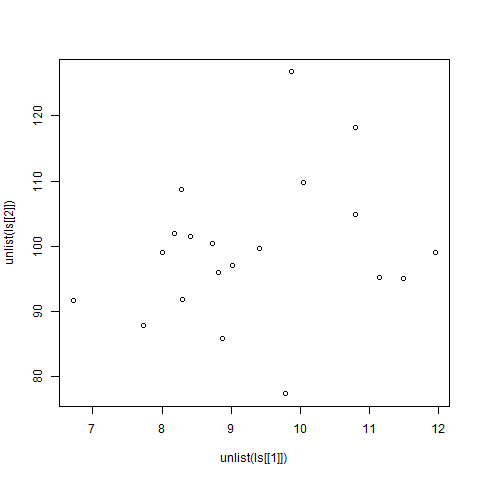# Plotting Graphs using Two Dimensional List in R Programming

List is a type of an object in R programming. Lists can contain heterogeneous elements like strings, numeric, matrices, or even lists. A list is a generic vector containing other objects. Two-dimensional list can be created in R programming by creating more lists in a list or simply, we can say nested lists. The `list()` function in R programming is used to create a list. In this article, we’ll learn to create plot graph using Two Dimensional List in R programming.

#### Creating a Two Dimensional list

A Two Dimensional list can be created with the use of `list()` function.

Syntax: list(x)

Parameter:
x: represents objects to be inserted in list

Example:

 `# Defining objects ` `x <``-` `c(``1``, ``2``, ``3``, ``4``) ` ` `  `y <``-` `LETTERS[``1``:``4``] ` ` `  `# Adding lists into a list ` `ls <``-` `list``( ` `  ``list``(x),  ` `  ``list``(y) ` `) ` ` `  `# Print list ` `print``(ls) `

Output:

```[]
[][]
 1 2 3 4

[]
[][]
 "A" "B" "C" "D"

```

#### Creating Plot Graph using Two Dimensional List

To create plot graphs, lists have to be passed as vectors to the `plot()` function as coordinate values. The `unlist()` function converts the list into an atomic type of vector.

Example:

 `# Creating nested lists with random values ` `ls <``-` `list``( ` `  ``list``(rnorm(``20``, mean ``=` `10``, sd ``=` `2``)), ` `  ``list``(rnorm(``20``, mean ``=` `100``, sd ``=` `10``))) ` ` `  `# Output to be present as PNG file ` `png(``file` `=` `"2DListGraph.png"``) ` ` `  `# Plotting list ` `plot(unlist(ls[[``1``]]), unlist(ls[[``2``]])) ` ` `  `# Saving the file ` `dev.off() `

Output:My Personal Notes arrow_drop_upBlockchain Enthusiast

If you like GeeksforGeeks and would like to contribute, you can also write an article using contribute.geeksforgeeks.org or mail your article to contribute@geeksforgeeks.org. See your article appearing on the GeeksforGeeks main page and help other Geeks.

Please Improve this article if you find anything incorrect by clicking on the "Improve Article" button below.

Article Tags :

Be the First to upvote.

Please write to us at contribute@geeksforgeeks.org to report any issue with the above content.